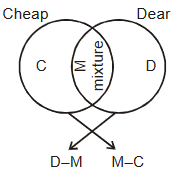# Mixture and Alligation

Pratice Mixture and Alligation Questions and answers.

## Importance of "Mixture and Alligation"

Mixture is a specific type of ratio and proportion, but since 1 or 2 questions from this chapter are asked regularly in competitions hence it is comfortable and useful to study this chapter separately. Questions are of limited nature hence marks can been sured with very less efforts.

## Mixture and Alligation - Scope of Questions

Questions are related to getting new mixture or alloy from mixing of two liquids/metals. In final mixture you have to find ratio of elements or to access required quantity to get a certain ratio.

## Mixture and Alligation - Way to Success

In these questions, it is very useful to know alligation method and other.

## Mixture and Alligation - Important Rules and Shortcut Tricks

Rule Description
1

The cost of cheap object is Rs. C/kg and the cost of dear object is Rs. D/kg. If the mixture of both object costs Rs. M/kg. then

$\frac{Cheap \ object}{Dear \ object}=\frac{D-M}{M-C}$Ratio is $(D – M) : (M–C)$

2

Quantity of x in mixture =

$\frac{Ratio \ of \ x \times Quantity \ of \ Mixture}{Sum \ of \ Ratios}$

3

If from x litre of liquid A, p litre is withdrawn and same quantity of liquid B is added. Again from mixture q litre mixture is withdrawn and same quantity of liquid B is added. Again from mixture, r litre is withdrawn and same quantity of liquid B is added, then

• In final mixture, liquid A is

$x\left(\frac{x-p}{q}\right)\left(\frac{x-q}{x}\right)\left(\frac{x-r}{x}\right).......$

• If only one process is repeated n times, then liquid A in final mixture is =

$x\left(\frac{x-p}{n}\right)^n$

• liquid B in final mixture =

x – (liquid A in final mixture)

4

If x is initial amount of liquid, p is the amount which is drawn, and this process is repeated n-times such that the resultant mixture is in the ratio a : b then,

$\frac{a}{a+b}=\left(\frac{x-p}{x}\right)^n$

5

There are two pots of same volume. Both the pots contains mixture of milk and water in the ratio m : n and p : q respectively. If both the mixtures are mixed together in a big pot, then what will be the final ratio of milk and water =

$\left(\frac{m}{m+n}+\frac{p}{p+q}\right):\left(\frac{n}{m+n}+\frac{q}{p+q}\right)$

6

The ratio of milk and water in the mixture of ‘x’ unit liquid is a : b. If ‘d’ unit milk is added to it then ratio of milk and water becomes a1 : b1. Then,

$d=\frac{x\left(a_1b-ab_1\right)}{\left(a+b\right)b_1}$

If ‘d’ unit water is added to it then,

$d=\frac{x\left(ab-a_1b_1\right)}{\left(a+b\right)a_1}$

7

There is x% milk in ‘a’ unit mixture of milk and water. The amount of milk that should be added to increase the percentage of milk from x% to y% is given by

Required quantity of milk =

$\frac{a\left(y-x\right)}{\left(100-y\right)}$

8

There is x% water in ‘a’ unit of the mixture of sugar and water. The quantity of water vapourised such that decrease in the percentage of water is from x% to y% is given by

Required quantity of vapourised water =

$\frac{a\left(x-y\right)}{y}$